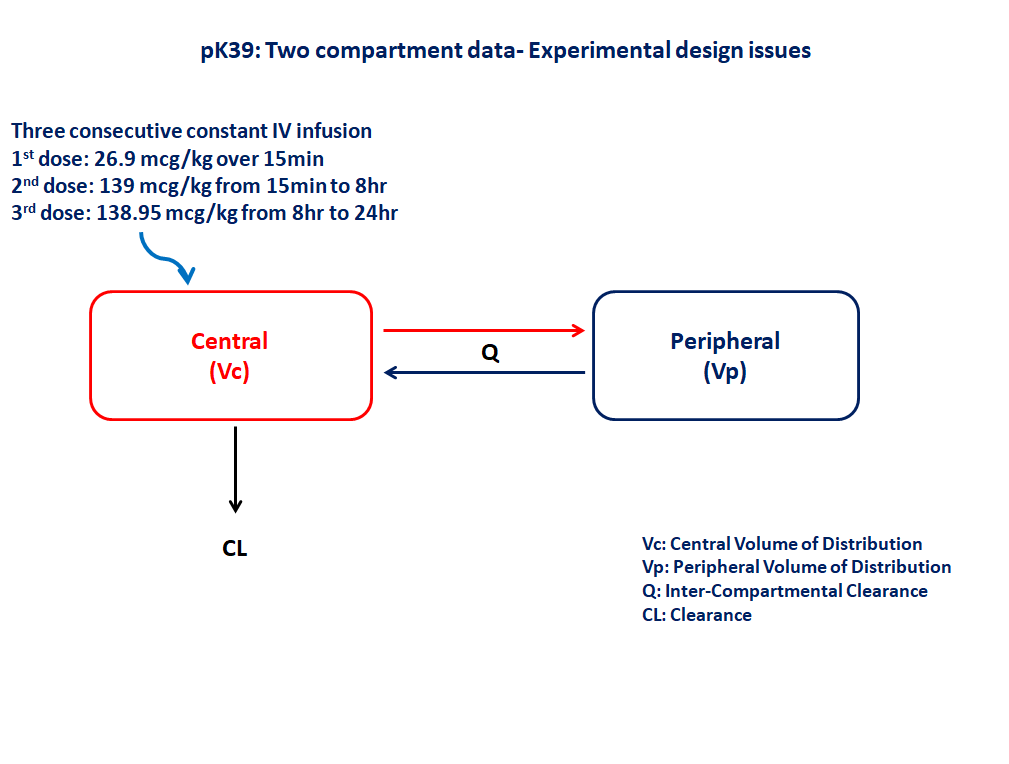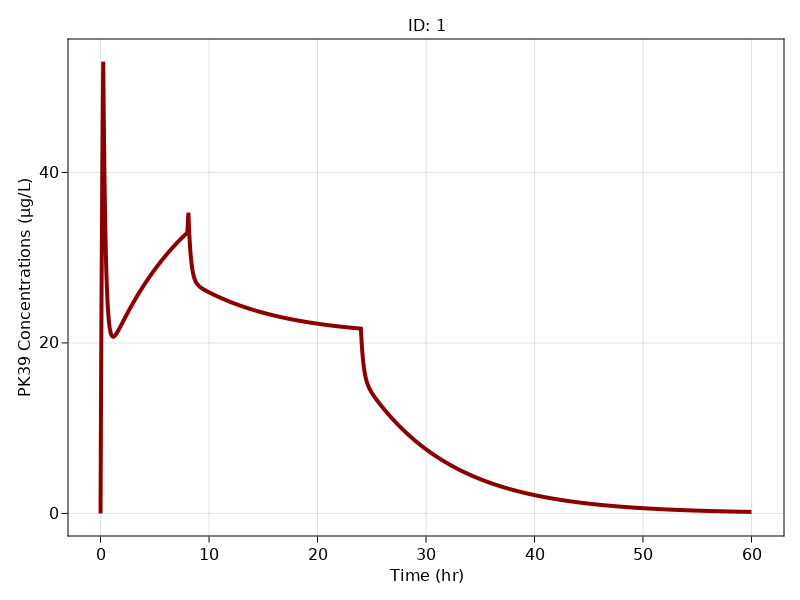# Exercise PK39 - Two Compartment data-Experimental design issues

### Background

• Structural model - Two compartment linear elimination with zero order absorption

• Route of administration - Three consecutive constant rate IV infusion

• Dosage Regimen - 1st dose:- 26.9 mcg/kg over 15min, 2nd dose:- 139 mcg/kg from 15min to 8hr, 3rd dose:- 138.95 mcg/kg between 8hr to 24hr

• Number of Subjects - 1### Objectives

In this model you will learn how to build a two compartment model and simulate for a single subject.

### Libraries

Call the "necessary" libraries to get start.

using Random
using Pumas
using PumasUtilities
using CairoMakie


### Model

In this two compartment model we administer three consecutive IV infusion for a single subject and we assess the disposition of drug and fitting the model to the observed data.

pk_39           = @model begin
desc        = "Two Compartment Model"
timeu       = u"hr"
end

@param begin
"Clearance (L/kg/hr)"
tvcl        ∈ RealDomain(lower=0)
"Volume of Central Compartment (L/kg)"
tvvc        ∈ RealDomain(lower=0)
"Volume of Peripheral Compartment (L/kg)"
tvvp        ∈ RealDomain(lower=0)
"Intercompartmental clearance (L/kg/hr)"
tvq         ∈ RealDomain(lower=0)
Ω           ∈ PDiagDomain(4)
"Proportional RUV"
σ²_prop     ∈ RealDomain(lower=0)
end

@random begin
η           ~ MvNormal(Ω)
end

@pre begin
CL          = tvcl * exp(η)
Vc          = tvvc * exp(η)
Vp          = tvvp * exp(η)
Q           = tvq * exp(η)
end

@dynamics begin
Central'    =  (Q/Vp)*Peripheral - (Q/Vc)*Central -(CL/Vc)*Central
Peripheral' = -(Q/Vp)*Peripheral + (Q/Vc)*Central
end

@derived begin
cp          = @. Central/Vc
"""
Observed Concentration (ug/L)
"""
dv          ~ @. Normal(cp, sqrt(cp^2*σ²_prop))
end
end

PumasModel
Parameters: tvcl, tvvc, tvvp, tvq, Ω, σ²_prop
Random effects: η
Covariates:
Dynamical variables: Central, Peripheral
Derived: cp, dv
Observed: cp, dv


### Parameters

Parameters provided for simulation. tv represents the typical value for parameters.

• $Cl$ - Clearance (L/kg/hr)

• $Vc$ - Volume of Central Compartment (L/kg)

• $Vp$ - Volume of Peripheral Compartment (L/kg)

• $Q$ - Intercompartmental clearance (L/kg/hr)

• $Ω$ - Between Subject Variability

• $σ$ - Residual error

param = (tvcl    = 0.417793,
tvvc    = 0.320672,
tvvp    = 2.12265,
tvq     = 0.903188,
Ω       = Diagonal([0.0,0.0,0.0,0.0]),
σ²_prop = 0.005)

(tvcl = 0.417793, tvvc = 0.320672, tvvp = 2.12265, tvq = 0.903188, Ω = [0.0
0.0 0.0 0.0; 0.0 0.0 0.0 0.0; 0.0 0.0 0.0 0.0; 0.0 0.0 0.0 0.0], σ²_prop =
0.005)


### Dosage Regimen

Single subject receiving three consecutive IV infusion

• 1st dose: 26.9 mcg/kg over 15min

• 2nd dose: 139 mcg/kg from 15min to 8hr

• 3rd dose: 138.95 mcg/kg between 8hr to 24hr

ev1  = DosageRegimen([26.9,139,138.95], time=[0,0.25,8], cmt=1, duration=[0.25,7.85,16])
sub1 = Subject(id=1, events=ev1)

Subject
ID: 1
Events: 6


### Simulation

Lets simulate for plasma concentration with the specific observation time points after IV infusion.

Random.seed!(123)
sim_sub1 = simobs(pk_39, sub1, param, obstimes=0:0.01:60)


### Visualization

f1, a1, p1 = sim_plot(pk_39, [sim_sub1],
observations = :cp,
color = :redsblues,
linewidth = 4,
axis = (
xlabel = "Time (hr)",
ylabel = "PK39 Concentrations (μg/L)",
xticks = 0:10:60))
f1